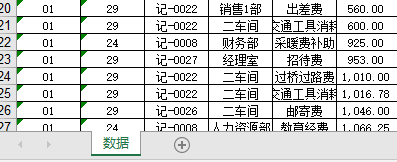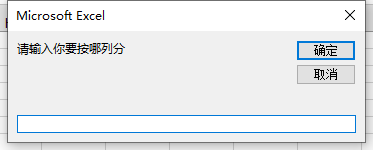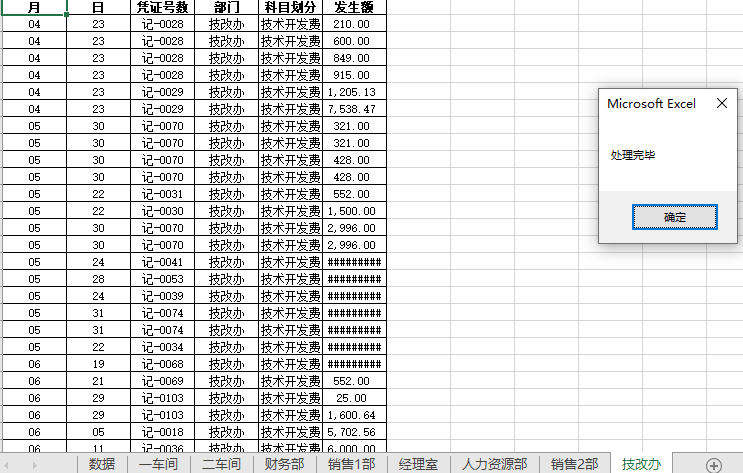• VBA 按照某一列进行筛选拆分数据，拆分成多个表或多个Sheet，按某列检查重复生成新的Sheet，删除除本表外的所有表
• VBA源代码，根据某列中的最大值，拿到该行的行号。此代码为取出若干小区的信息，7*24小时，拿到某列值最大的行号，精简成7行
• Vba获取表格某一列的值，判断单元格是否为空，是则开始计数并返回
• Sub d() Dim i As Long Dim r As Long i = 2 r = 2 Do While r <= Sheet2.Rows.Count 'Range("K1").EntireColumn.Cells(i, 1).Value = Range("F2").EntireColumn.Cells(r, 1).Value ...
Sub d()

Dim i As Long
Dim r As Long
i = 2
r = 2
Do While r <= Sheet2.Rows.Count

'Range("K1").EntireColumn.Cells(i, 1).Value = Range("F2").EntireColumn.Cells(r, 1).Value
Range("L1").EntireColumn.Cells(i, 1).Value = r - 1
Range("M1").EntireColumn.Cells(i, 1).Value = Range("H2").EntireColumn.Cells(r, 1).Value
i = i + 1
r = r + 25
Loop
End Sub

展开全文vba EXCEL
• Debug.Print Worksheets(1).[A65536].End(xlUp).Row ...功能是返回个 Range 对象，该对象代表包含源区域的区域尾端的单元格。等同于按键 End+ 向上键、End+ 向下键、End+ 向左键或 End+ 向右键。Range 对象，只读。

Debug.Print Worksheets(1).[A65536].End(xlUp).Row

完整介绍Excel End(xlUp).Row

一、End(xlUp).Row介绍

功能是返回一个 Range 对象，该对象代表包含源区域的区域尾端的单元格。等同于按键 End+ 向上键、End+ 向下键、End+ 向左键或 End+ 向右键。Range 对象，只读。

其语法如下：

expression.End(Direction)

（本文来源于图老师网站，更多请访问http://www.tulaoshi.com）

expression 必需。 该表达式返回应用于列表中的对象之一。

Direction XlDirection 类型，必需。所要移动的方向。

XlDirection 可为 XlDirection 常量之一。

xlDown

xlToRight

xlToLeft

xlUp

二、End(xlUp).Row示例

本示例选定包含单元格 B4 的区域中 B 列顶端的单元格。

Range("B4").End(xlUp).Select

本示例选定包含单元格 B4 的区域中第 4 行尾端的单元格。

Range("B4").End(xlToRight).Select

本示例将选定区域从单元格 B4 延伸至第四行最后一个包含数据的单元格。

Worksheets("Sheet1").Activate

（本文来源于图老师网站，更多请访问http://www.tulaoshi.com）

Range("B4", Range("B4").End(xlToRight)).Select

再比如：

Range("a65536").End(xlUp).Row A列数据区域最后一行的行号

Range("b65536").End(xlUp).Row B列数据区域最后一行的行号

Range("d65536").End(xlUp).Row D列数据区域最后一行的行号

Range("k65536").End(xlUp).Row K列数据区域最后一行的行号

最后，再看看一段VBA调用的代码吧：

Dim i, M, iRow, sh, arr

M = 0.0015

For Each sh In ThisWorkbook.Sheets

（本文来源于图老师网站，更多请访问http://www.tulaoshi.com）

iRow = sh.[D65535].End(xlUp).Row

ReDim arr(1 To iRow, 1 To 2)

arr = sh.Range("D3:E" & iRow)

For i = 2 To UBound(arr)

arr(i, 1) = arr(i, 1) * M

arr(i, 2) = arr(i, 2) * M

Next

arr(1, 1) = Application.Substitute(arr(1, 1), "平方米", "亩")

arr(1, 2) = Application.Substitute(arr(1, 2), "平方米", "亩")

sh.Range("D3:E" & iRow) = arr

Next

展开全文• 根据自定义的某一列创建工作表项目分析需求分析解决思路及代码最终效果图知识点总结整体代码 项目分析 项目所在地址 位置：王佩丰 VBA 课件\第七课 需求分析 在处理财务数据时，可能需要根据某一行中的数据对整个...

项目分析

项目所在地址
位置：王佩丰 VBA 课件\第七课

需求分析

在处理财务数据时，可能需要根据某一行中的数据对整个工作表进行分类创建各自的工作表

待处理的表格解决思路及代码

1、对整个excel进行分析，判断是否存在一些无意义的工作表
（这里只是为了让最终生成的工作表只有我们需要的），若有，则删除

If Sheets.Count > 1 Then

'For g = 2 To Sheets.Count

'Sheets(g).Delete
'Next
For Each sht In Sheets
If sht.Name <> "数据" Then
sht.Delete
End If
Next

End If

2、根据我们选中的列去创建所有类别的工作表，此步需要遍历每一行的数据。

For i = 2 To row_number
k = False

For j = 1 To Sheets.Count
If Sheet1.Cells(i, l).Value = Sheets(j).Name Then
k = True
Exit For
End If
Next

If k = False Then
'创建表格
Sheets(Sheets.Count).Name = Sheet1.Cells(i, l).Value
'复制第一行数据
'Sheet1.Range("a1").EntireRow.Copy Sheets(Sheets.Count).Range("a1")
End If

'Sheet1.Range("a" & i).EntireRow.Copy Sheets(Sheet1.Range("d" & i).Value).Range("a" & Sheets(Sheet1.Range("d" & i).Value).Range("a65535").End(xlUp).Row + 1)

Next

3、采用筛选功能，将某一类别的数据筛选出来并复制到其所对应的工作表内。

For j = 2 To Sheets.Count
Sheet1.Range("a1:f" & row_number).AutoFilter Field:=l, Criteria1:=Sheets(j).Name
Sheet1.Range("a1:f" & row_number).Copy Sheets(j).Range("a1")

Next
Sheet1.Range("a1:f" & row_number).AutoFilter

最终效果图

1、先选择列数2、运行结果知识点总结

1、对于输入弹框只需要一下代码，注意其输入值可以赋值给变量，并且inputbox后需要添加括号

l = InputBox("请输入你要按哪列分")

2、在删除无意义的工作表时，不能采用for循环而是用For each，
采用for循环时，会出现越界的问题，
这是因为当时你删除其中一个表格后，其后边表格数会减少即sheet2变成sheet1，最终删不干净。
4、由于该项目中列数也变成的变量，
故在选择表格时，不能再使用Range,而是cells,原因如下：

选择工作表中表格的方法：

方法解释
Range(“a1”).select这里的行可以采用变量的形式，而列是采用字母表示不能采用变量
Cells(2，1).select这里选中的是第一行第二列，行号和列号均可以采用变量表示

5、下面展示 弹框代码

MsgBox "处理完毕"

整体代码

Sub shi()

Dim i, j, row_number, g As Integer
Dim k As Boolean
Dim l As Integer
Dim sht As Worksheet

l = InputBox("请输入你要按哪列分")

row_number = Sheet1.Range("a65535").End(xlUp).Row

'删除无意义的表
If Sheets.Count > 1 Then

'For g = 2 To Sheets.Count

'Sheets(g).Delete
'Next
For Each sht In Sheets
If sht.Name <> "数据" Then
sht.Delete
End If
Next

End If

For i = 2 To row_number
k = False

For j = 1 To Sheets.Count
If Sheet1.Cells(i, l).Value = Sheets(j).Name Then
k = True
Exit For
End If
Next

If k = False Then
'创建表格
Sheets(Sheets.Count).Name = Sheet1.Cells(i, l).Value
'复制第一行数据
'Sheet1.Range("a1").EntireRow.Copy Sheets(Sheets.Count).Range("a1")
End If

'Sheet1.Range("a" & i).EntireRow.Copy Sheets(Sheet1.Range("d" & i).Value).Range("a" & Sheets(Sheet1.Range("d" & i).Value).Range("a65535").End(xlUp).Row + 1)

Next

For j = 2 To Sheets.Count
Sheet1.Range("a1:f" & row_number).AutoFilter Field:=l, Criteria1:=Sheets(j).Name
Sheet1.Range("a1:f" & row_number).Copy Sheets(j).Range("a1")

Next
Sheet1.Range("a1:f" & row_number).AutoFilter

MsgBox "处理完毕"

Sheet1.Select

End Sub

展开全文excel
• 首先，将需要拆分的sheet命名为“明细”，接下来运行此代码，按提示操作即可。 在这里插入代码片 Sub chaifen() '定义变量类型 Dim sht, sh1, sh2 As Worksheet ...'程序开始是要求输入按哪一列拆分数...

首先，将需要拆分的sheet命名为“明细”，接下来运行此代码，按提示操作即可。

在这里插入代码片
Sub chaifen()
'定义变量类型
Dim sht, sh1, sh2 As Worksheet
Dim k, i, j As Integer
Dim irow As Integer
Dim col As Integer
Dim str As String

'程序开始是要求输入按哪一列拆分数据
col = InputBox("请输入你要按哪一列拆分数据")

'获取所选择的文件夹路径
Set fileDlg = Application.FileDialog(msoFileDialogFolderPicker)

With fileDlg

If .Show = -1 Then

For Each fld In .SelectedItems

str = fld

Next fld

End If

End With

Application.ScreenUpdating = False '防止屏幕一直闪动

'开始时先删除无意义的表，只留下需要拆分的sheet

If Sheets.Count > 1 Then
For Each sht1 In Sheets
If sht1.Name <> "明细" Then
sht1.Delete
End If
Next
End If

'拆分明细这张sheet
irow = Sheet1.Range("a1048576").End(xlUp).Row '用于计算sheet1一共有几行
For i = 2 To irow
k = 0
For Each sht In Sheets
If sht.Name = Sheet1.Cells(i, col) Then
k = 1
End If
Next

If k = 0 Then
Sheets(Sheets.Count).Name = Sheet1.Cells(i, col)
End If
Next
'拷贝数据到“明细”后面的sheet2,sheet3,sheet4....中
For j = 2 To Sheets.Count
Sheet1.Range("a1:s" & irow).AutoFilter Field:=col, Criteria1:=Sheets(j).Name
Sheet1.Range("a1:s" & irow).Copy Sheets(j).Range("a1")
Next

Sheet1.Range("a1:s" & irow).AutoFilter '取消筛选
Sheet1.Select

'将其中的sheets拆分为多个Excel文件

For Each sht2 In Sheets
If sht2.Name <> "明细" Then
sht2.Copy
ActiveWorkbook.SaveAs Filename:=str & "\" & sht2.Name & ".xlsx"
ActiveWorkbook.Close
End If
Next

Application.ScreenUpdating = True
MsgBox "已处理完毕"

End Sub

展开全文Excel VBA
• VBA判断某列数据重复存在

千次阅读 2017-07-22 21:59:05
如果数据重复存在，则将重复数据标红色并打印到另一列vba
• Sub 保留表头拆分数据为若干新工作簿()  Dim arr, d As Object, k, t, i&amp;, lc%, rng As Range, c% ...请输入拆分号", , 4, , , , , 1)  If c = 0 Then Exit Sub  Application.ScreenUpdating = ...
• Sub test() Dim arr() As String '定义动态数组 Dim n As Long Dim i As Integer n = Application.WorksheetFunction.CountA(Range("A:A")) '确定A非空数据数量 ReDim arr(1 To n) As Stringexcel vba
• "Cells(Rows.Count, 1)"表示是查找A最后个非空单元格，按的可以改成"Cells(1, Columns.count)" "end(3)"表示的向上查找，也可以写成“end(xlup)”,还有其他3个方向，向下，向左，向右：xldown,xltoleft,...excel vba
• 背景 业务需要依据详细设计文档编写所有对应的页面 问题 工作量大，11张大表（几十上百字段）时间紧 3天完成 核心 有完整的表设计，可通过excel手动编辑完成...通过代码实现手工操作excel的工作，也就是编写VBA宏 ...
• vba隐藏，显示列

千次阅读 2020-04-17 10:24:54
2个页面(首页、数据库整理)，在首页中D填写1来选择数据库整理页面中要隐藏的。首页中C内容通过超链接连接到数据库整理页面的对应中。 支持选择性隐藏；显示隐藏的；隐藏所有的 '隐藏 Sub ...vba excel
• VBA删掉某列中含有空值的行

千次阅读 2020-06-09 16:44:31
Sub Del() num = ActiveSheet.UsedRange.Rows.Count() For i = 1 To num If Cells(i, 9) = "" Then Rows(i).Delete End If Next End Sub
• 假如某一列包含多种重复单元，但是重复的单元对应的其它列却不相同，需求便是指定某一列，寻找其中相同的元素，对两行元素进行合并。如下图所示的源数据， 可以看到，该Excel表格的A列有很多重复项，对他们进行合并...
• Dim sj(), sj1, sj2, jg(), cnt&, d&, h&, hh&, k&, l&, m&, n&, nn&, p&, q& Sub kagawa() tms = Timer d = [h3]: l = [h6]: If l = 0 Then l = 65535 h = [h1] * 10 ^ d: hh = [h2] * 10 ^ d: If hh >excel vba
• 在处理php数组的时候，有种需求特别的频繁，如下二维数组： $arr = array( 1=>array( 'id' => 5, 'name' => '张三' ), 2=>array( 'id' => 6, 'name' => '李四' ) ); 目的就是要取到key为name的集合，得到这个结果... • Sub chaifenshuju() Dim sht As Worksheet Dim k, i, j As Integer Dim irow As Integer ...l = InputBox("请输入你要按哪分") '删除无意义的表 Application.DisplayAlerts = False If Sheets.Count &...vba excel • 笔者最近在做个数据库项目，其中需要从EXCEL中提取关键字段。提取内容如下图所示，需要将图中加粗部分单独提出后进行去噪处理。如果通过word处理，文字量小的时候尚可实现，但几十万字的处理量很容易就造成假死。... • vba遍历列数据 千次阅读 2020-10-09 14:10:13 Sub funcff() Dim i, j, l, o, d, h l = Columns.Count l = 21 j = 2 o = 22 d = 4 h = 20 For i = 1 To l Cells(h, j).Select j = j + 7 Selection.Copy Cells(o, 1).Select ActiveSheet.Paste ...o = o + • '筛选某列包含OK,不包含12的单元格 Function Filter(rag As Range) With CreateObject("VBscript.regexp") rag = Worksheets("sheet1").Range("B1:B6666"). .Global = True .Pattern = "(.*[OK])(?!.*)" If...vba • 前几天放假回家，父亲让我写一个可以对EXCEL进行操作的小demo：通过某一列的值寻找该值所在行的所有内容，且复制到另一张表中。 感觉很简单，就答应了 考虑到电脑环境、操作性、简易性等。我决定用VBA来写。毕竟是...excel vba • Excel VBA获取最后一行列 万次阅读 2015-11-04 21:17:31 VBA代码： Function getLastRow() Debug.Print "End(xlUp):" & Sheets(1).[A65536].End(xlUp).Row Debug.Print "usedRange:" & ActiveSheet.UsedRange.Rows.Count Debug.Print "findExcel vba • excel VBA获取EXCEL中的行数和数. • VBA 中 是一列单元格 不可编辑 而其他的可以编辑 就是查询出来的数据主键那一列 我想让那一列不能编辑 只能看 应该怎么写代码excel vba 数据 • SQL SERVER将某一列字段中的某个值替换为其他的值 update 表名 set 列名 = REPLACE( 列名 ,’aa’,’bb’) SQL SERVER”函数 replace 的参数 1 的数据类型 ntext 无效”解决办法 UPDATE 表名 SET 列名= REPLACE(CAST... • 暂时只实现了删除一列中的空行，并没有实现多行的判断空行和删除方法。----之后再做更复杂的 1.1需求分析 用VBA删除如下内容，解决思路都不同 删除1列的空行（本文要做的） 删除整个区域内的空行 删除整个... • 这个例子是对课程进度表里面的某个学生的剩余课时进行求和汇总。 laravel 版本是 lts 5.5 StudentLessonProgress::where('student_info_id',$student_info_id) ->sum('total_left_class_num');...
• 在Excel中，经常需要得知某列最后个非空单元格的行号，以确定数据的数量。编写自定义函数以实时计算单元格所在的最后非空单元格的行号。 员工编号 姓名 部门 0114 俞卫广 总经办 0374 ...
• How do I find the number of used columns in an Excel sheet using VBA?Dim lastRow As LonglastRow = Sheet1.Range("A" & Rows.Count).End(xlUp).RowMsgBox lastRowUsing the above VBA I'm able to find the......